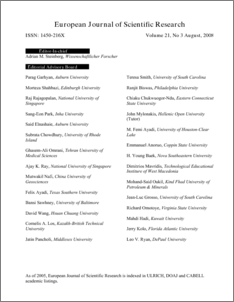# Variable Step Variable Order Two Point Block Fully Implicit Method for Solving Ordinary Differential Equations

## Citation

Abdul Majid, Zanariah and Suleiman, Mohamed (2008) Variable Step Variable Order Two Point Block Fully Implicit Method for Solving Ordinary Differential Equations. European Journal of Scientific Research, 21 (3). pp. 521-529. ISSN 1450-216X

## Abstract

The aim of this paper is to investigate the performance of the developed two point block methods of order 5, 7 and 9 for solving first order Ordinary Differential Equations (ODEs) using variable step size and order. The code will combine three proposed block methods i.e the 2-point one block fully implicit block method of order 5, the 2-point two block fully implicit block method of order 7 and the 2-point three block fully implicit block method of order 9. These methods will estimate the numerical solution at two equally spaced points simultaneously within a block. The existence multistep method involves the computations of the divided differences and integration coefficients when using the variable step size or variable step size and order. The block method developed will be presented in the simple form of the Adams Moulton type. The performances of the code will be compared in terms of maximum error, total number of steps and execution times with the existence non block method and 2-point block method of variable step size and order code.Preview
PDF
139.pdfView Item